Related Articles

# Class 9 NCERT Solutions – Chapter 13 Surface Areas And Volumes – Exercise 13.3

• Last Updated : 04 Mar, 2021

### Question 1: Diameter of the base of a cone is 10.5 cm and its slant height is 10 cm. Find its curved surface area.

Solution:

Given:

Attention reader! All those who say programming isn't for kids, just haven't met the right mentors yet. Join the  Demo Class for First Step to Coding Coursespecifically designed for students of class 8 to 12.

The students will get to learn more about the world of programming in these free classes which will definitely help them in making a wise career choice in the future.

Slant height of cone, (l) = 10 cm

Since, Curved surface area of cone is = πrl

= (22/7)×5.25×10 = 165 cm2

Therefore, the curved surface area of the cone of height 10m and base 10.5 cm is 165 cm2.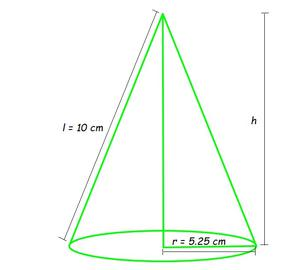### Question 2: Find the total surface area of a cone, if its slant height is 21 m and diameter of its base is 24 m.

Solution:

Given:

Radius (r) = 24/2 m = 12m

Slant height, (l) = 21 m

Since, Total surface area of the cone = πr(l+r)

= (22/7)×12×(21+12) m2

= 1244.57m2

Therefore, the total surface area of the cone with height 21m and base 24m is 1244.57m2.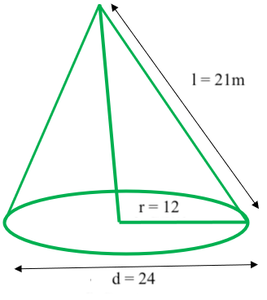### (ii) total surface area of the cone.

Solution:

Given:

Slant height (l) = 14 cm

Curved surface area = 308 cm2

Curved surface area = 308 cm2

Curved surface area of cone = πrl

(308) = (22/7)×r×14

308 = 44 r

r = 308/44 = 7

Radius of a cone base is 7 cm.

(ii) total surface area of the cone.

Total surface area of cone = πr(l+r)

Total surface area of cone = 308+(22/7)×72

= 308+154

= 462 cm2

Therefore, the total surface area of the cone with CSA as 308 cm2 is 462 cm2.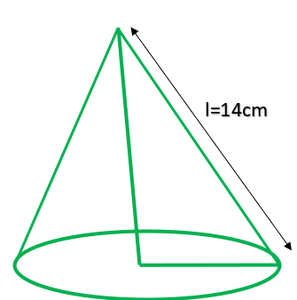### (ii) cost of the canvas required to make the tent, if the cost of 1 m2 canvas is Rs. 70.

Solution:

Given:

Height of conical tent (h) = 10m

Radius of conical base (r) = 24m

Slant height of the tent = l.

(i) slant height of the tent.

l2 = h2 + r2    [using Pythagoras theorem]

= (10)2 + (24)2

= 676

l = 26

Therefore, the slant height of the tent = 26 m.

(ii) cost of the canvas required to make the tent, if the cost of 1 m2 canvas is Rs. 70.

Curved surface area of tent = πrl

= (22/7) × 24 × 26 m2

As cost of 1 m2 canvas = Rs 70

Cost of (13728/7)m2 canvas = (13728/7)×70

= Rs 137280

Therefore, the cost of the canvas required to make the tent is Rs 137280.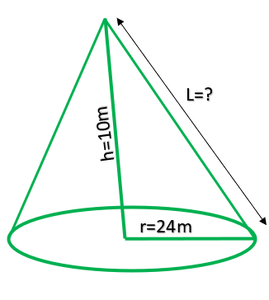### Question 5: What length of tarpaulin 3 m wide will be required to make conical tent of height 8 m and base radius 6 m? Assume that the extra length of material that will be required for stitching margins and wastage in cutting is approximately 20 cm (Use π = 3.14).

Solution:

Height of conical tent (h) = 8m

Radius of base of tent (r) = 6m

Slant height of tent, l2 = r2+h2

l2 = 62+82

= 36+64

= 100 [Taking square root on both the side]

l = 10

As, Curved surface area = πrl

= (3.14×6×10) m2

= 188.4m2

Let the length of tarpaulin sheet required = L.

As 20 cm will be wasted,

Therefore, the Effective length will be (L – 0.2m).

Given breadth of tarpaulin = 3m

Area of sheet = Curved surface area of tent

[(L – 0.2) × 3] = 188.4

L – 0.2 = 62.8

L = 63 m

Therefore, the length of the required tarpaulin sheet will be 63 m.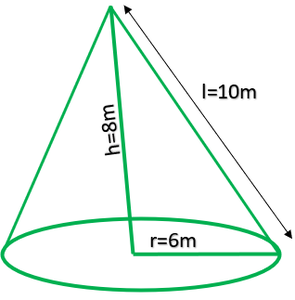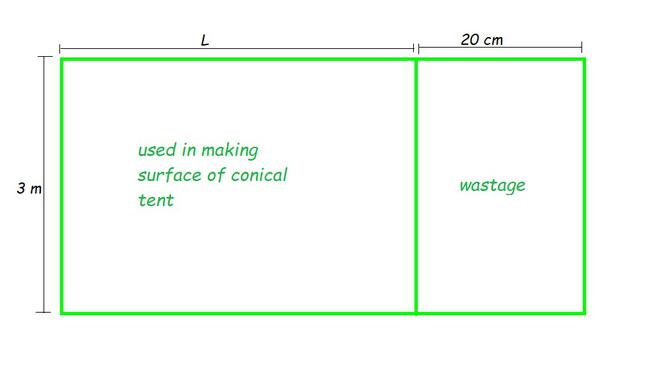### Question 6: The slant height and base diameter of a conical tomb are 25 m and 14 m respectively. Find the cost of white-washing its curved surface at the rate of Rs 210 per 100 m2.

Solution:

Given:

Slant height of conical tomb (l) = 25m

= 14/2 m

= 7m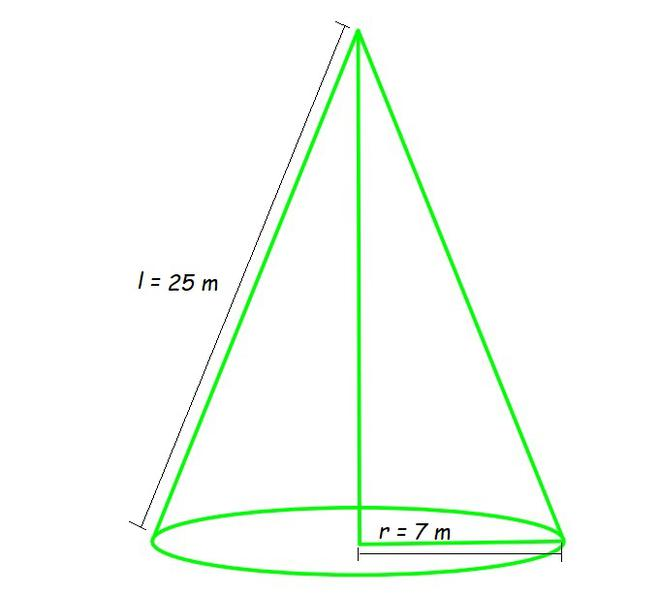Curved surface area of conical tomb = πrl

= (22/7)×7×25

= 550

Curved surface area of conical tomb= 550m2

Cost of white-washing 100 m2 area = Rs 210

Cost of white-washing 550 m2 area = Rs (210×550)/100

= Rs. 1155

Therefore, the cost will be Rs. 1155 while white-washing tomb of area 550 m2.

### Question 7: A joker’s cap is in the form of a right circular cone of base radius 7 cm and height 24 cm. Find the area of the sheet required to make 10 such caps.

Solution:

Given:

Radius of conical cap (r) = 7 cm

Height of conical cap (h) = 24cm

Slant height, l2 = r2+h2

= 72+242

= 49+576

= 625 [Taking square root on both the side]

l = 25 cm

Curved surface area of 1 conical cap = πrl

= (22/7)×7×24

= 550

Curved surface area of 10 caps = (10×550) cm2 = 5500 cm2

Therefore, the area of 5500 cm2 of the sheet is required to make 10 such caps.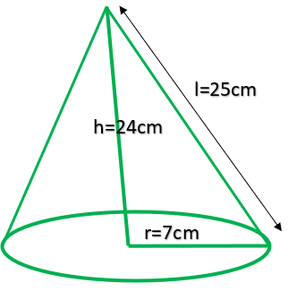### Question 8: A bus stop is barricaded from the remaining part of the road, by using 50 hollow cones made of recycled cardboard. Each cone has a base diameter of 40 cm and height 1 m. If the outer side of each of the cones is to be painted and the cost of painting is Rs 12 per m2, what will be the cost of painting all these cones? (Use π = 3.14 and take1.04 = 1.02)

Solution:

Given:

Radius of cone (r) = diameter/2

= 40/2 cm

= 20cm

= 0.2 m

Height of cone (h) = 1m

Slant height, l2 = r2 + h2

Using given values, l2 = (0.22+12)

= (1.04)

l = 1.02

Slant height of the cone (l) = 1.02 m

Now,

Curved surface area of each cone = πrl

= 3.14×0.2×1.02

= 0.64056

Curved surface area of 50 such cones = (50×0.64056) = 32.028

Curved surface area of 50 such cones = 32.028 m2

Also given Cost of painting 1 m2 area = Rs 12

Cost of painting 32.028 m2 area = Rs (32.028×12)

= Rs. 384.336

= Rs.384.34 (approximately)

Therefore, the cost of painting all the cones is Rs. 384.34.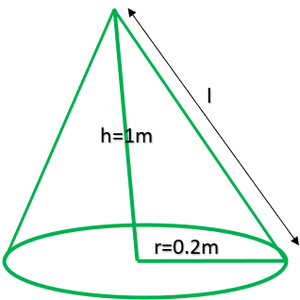My Personal Notes arrow_drop_up